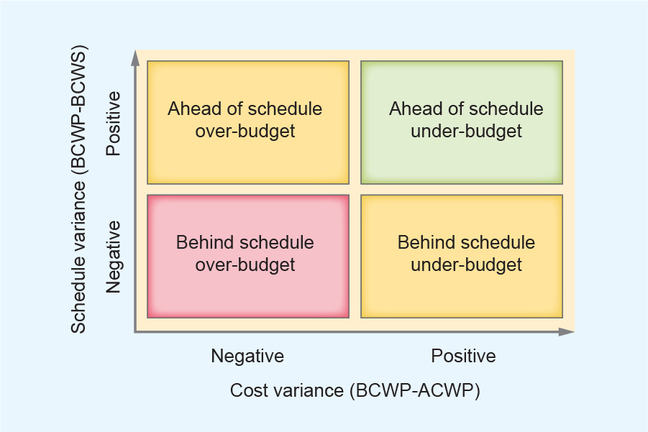## Want to keep learning?

This content is taken from the The Open University & The Open University Business School's online course, Business Fundamentals: Project Management. Join the course to learn more.
4.5

# Calculate schedule and cost variances

Two variances must be calculated in order to evaluate a project: the schedule variance and the cost variance.

We now know these data about the project:

• BCWS = £430
• BCWP = £365
• ACWP = £450

Schedule variance is defined as BCWP – BCWS. It is a comparison of the amount of work performed during a given period of time with what was scheduled to be performed. A negative variance means the project is behind schedule. The example project is therefore behind schedule:

${\rm{Schedule\ variance}} = £365 – £430 = £-65$

Cost variance is defined as BCWP – ACWP. It is a comparison of the budgeted cost of work performed with the actual costs to that moment. A negative variance means the project is over budget. The example project is therefore over budget:

${\rm{Cost\ variance}} = £365 – £450 = £-85$

Now, the project can be represented in this matrix:This matrix is a great tool to communicate the condition of your project to any stakeholder. The example project is in the worst condition: it is late and over-budget.

Project performance can also be calculated using the Schedule Performance Index (SPI) and the Cost Performance Index (CPI). For the SPI, an index of 1 is on schedule and an index less than 1 is behind schedule. For the CPI, an index of 1 indicates the project is on budget and an index less than 1 indicates an overspend against budget.

$\rm{SPI} = \rm{BCWP}/\rm{BCWS} = £365/£430 = 0.85$ $\rm{CPI} = \rm{BCWP}/\rm{ACWP} = £365/£450 = 0.81$

The Cost Schedule Index (CSI) is defined as SPI × CPI and is a measurement of the overall efficiency of the project. The more the CPI is below 1, the harder will be to recover the project. In the example project, the has a CSI of is 0.69. The Project value company event example file below shows you how to make these calculations.